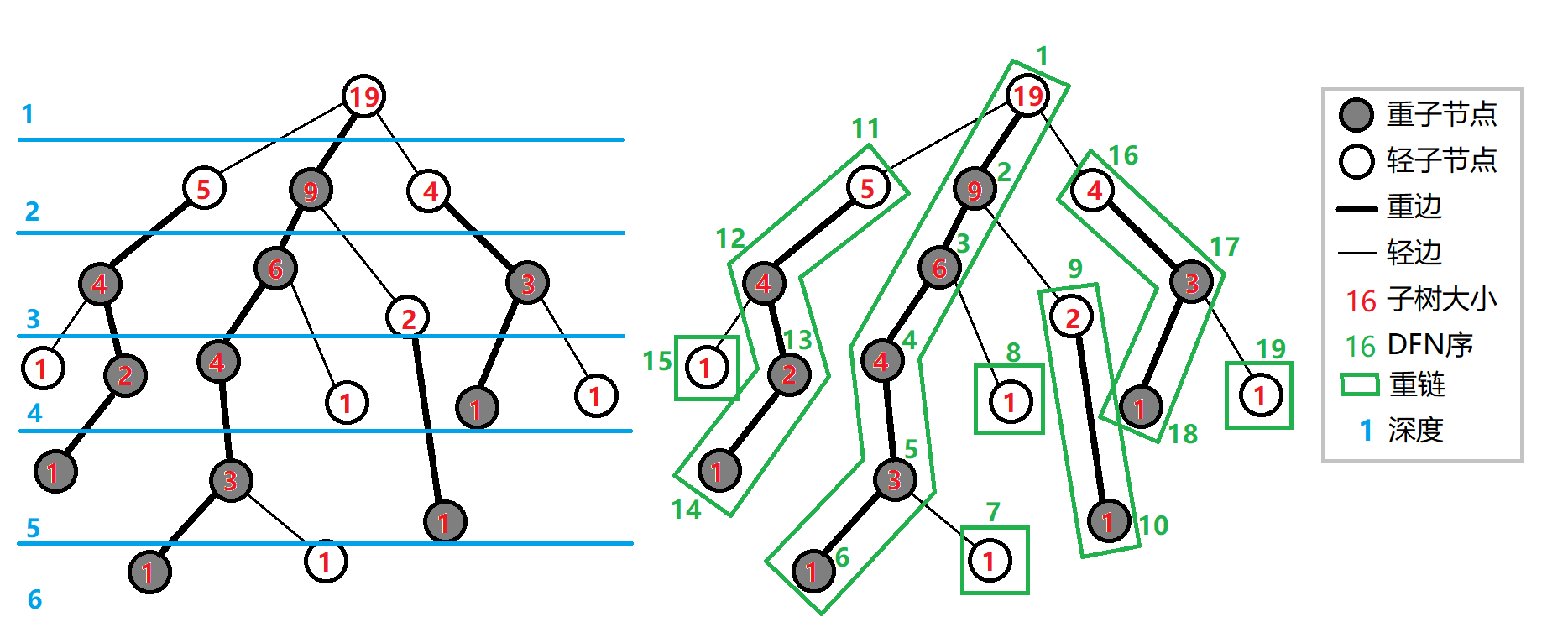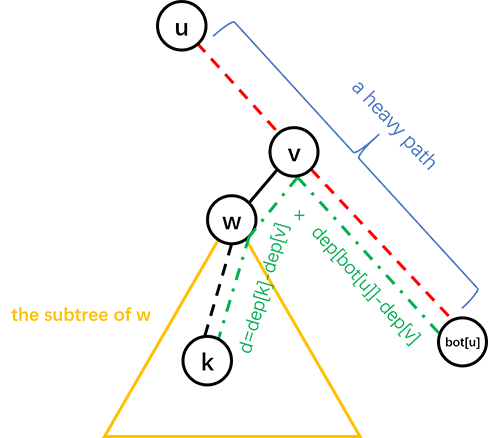# 树链剖分

## 树链剖分的思想及能解决的问题

1. 修改 树上两点之间的路径上 所有点的值。
2. 查询 树上两点之间的路径上 节点权值的 和/极值/其它（在序列上可以用数据结构维护，便于合并的信息）

## 重链剖分## 实现

• 表示节点 在树上的父亲。
• 表示节点 在树上的深度。
• 表示节点 的子树的节点个数。
• 表示节点 重儿子
• 表示节点 所在 重链 的顶部节点（深度最小）。
• 表示节点 DFS 序，也是其在线段树中的编号。
• 表示 DFS 序所对应的节点编号，有

  1 2 3 4 5 6 7 8 9 10 11 12 13 14 15 16 17 18 19 20 21 22 23 void dfs1(int o) { son[o] = -1; siz[o] = 1; for (int j = h[o]; j; j = nxt[j]) if (!dep[p[j]]) { dep[p[j]] = dep[o] + 1; fa[p[j]] = o; dfs1(p[j]); siz[o] += siz[p[j]]; if (son[o] == -1 || siz[p[j]] > siz[son[o]]) son[o] = p[j]; } } void dfs2(int o, int t) { top[o] = t; cnt++; dfn[o] = cnt; rnk[cnt] = o; if (son[o] == -1) return; dfs2(son[o], t); // 优先对重儿子进行 DFS，可以保证同一条重链上的点 DFS 序连续 for (int j = h[o]; j; j = nxt[j]) if (p[j] != son[o] && p[j] != fa[o]) dfs2(p[j], p[j]); } 

## 常见应用

### 求最近公共祖先

 1 2 3 4 5 6 7 8 9 int lca(int u, int v) { while (top[u] != top[v]) { if (dep[top[u]] > dep[top[v]]) u = fa[top[u]]; else v = fa[top[v]]; } return dep[u] > dep[v] ? v : u; } 

## 例题

### 「ZJOI2008」树的统计

#### 题目大意

1. 修改单个节点的权值；
2. 查询 的路径上的最大权值；
3. 查询 的路径上的权值之和。

#### 解法

1. 单点修改；
2. 区间查询最大值；
3. 区间查询和。

  1 2 3 4 5 6 7 8 9 10 11 12 13 14 15 16 17 // st 是线段树结构体 int querymax(int x, int y) { int ret = -inf, fx = top[x], fy = top[y]; while (fx != fy) { if (dep[fx] >= dep[fy]) ret = max(ret, st.query1(1, 1, n, dfn[fx], dfn[x])), x = fa[fx]; else ret = max(ret, st.query1(1, 1, n, dfn[fy], dfn[y])), y = fa[fy]; fx = top[x]; fy = top[y]; } if (dfn[x] < dfn[y]) ret = max(ret, st.query1(1, 1, n, dfn[x], dfn[y])); else ret = max(ret, st.query1(1, 1, n, dfn[y], dfn[x])); return ret; } 

  1 2 3 4 5 6 7 8 9 10 11 12 13 14 15 16 17 18 19 20 21 22 23 24 25 26 27 28 29 30 31 32 33 34 35 36 37 38 39 40 41 42 43 44 45 46 47 48 49 50 51 52 53 54 55 56 57 58 59 60 61 62 63 64 65 66 67 68 69 70 71 72 73 74 75 76 77 78 79 80 81 82 83 84 85 86 87 88 89 90 91 92 93 94 95 96 97 98 99 100 101 102 103 104 105 106 107 108 109 110 111 112 113 114 115 116 117 118 119 120 121 122 123 124 125 126 127 128 129 130 131 132 133 134 135 136 137 138 139 140  #include #include #include #define lc o << 1 #define rc o << 1 | 1 const int maxn = 60010; const int inf = 2e9; int n, a, b, w[maxn], q, u, v; int cur, h[maxn], nxt[maxn], p[maxn]; int siz[maxn], top[maxn], son[maxn], dep[maxn], fa[maxn], dfn[maxn], rnk[maxn], cnt; char op; inline void add_edge(int x, int y) { // 加边 cur++; nxt[cur] = h[x]; h[x] = cur; p[cur] = y; } struct SegTree { int sum[maxn * 4], maxx[maxn * 4]; void build(int o, int l, int r) { if (l == r) { sum[o] = maxx[o] = w[rnk[l]]; return; } int mid = (l + r) >> 1; build(lc, l, mid); build(rc, mid + 1, r); sum[o] = sum[lc] + sum[rc]; maxx[o] = std::max(maxx[lc], maxx[rc]); } int query1(int o, int l, int r, int ql, int qr) { // 查询 max if (l > qr || r < ql) return -inf; if (ql <= l && r <= qr) return maxx[o]; int mid = (l + r) >> 1; return std::max(query1(lc, l, mid, ql, qr), query1(rc, mid + 1, r, ql, qr)); } int query2(int o, int l, int r, int ql, int qr) { // 查询 sum if (l > qr || r < ql) return 0; if (ql <= l && r <= qr) return sum[o]; int mid = (l + r) >> 1; return query2(lc, l, mid, ql, qr) + query2(rc, mid + 1, r, ql, qr); } void update(int o, int l, int r, int x, int t) { // 更新 if (l == r) { maxx[o] = sum[o] = t; return; } int mid = (l + r) >> 1; if (x <= mid) update(lc, l, mid, x, t); // 左右分别更新 else update(rc, mid + 1, r, x, t); sum[o] = sum[lc] + sum[rc]; maxx[o] = std::max(maxx[lc], maxx[rc]); } } st; void dfs1(int o) { son[o] = -1; siz[o] = 1; for (int j = h[o]; j; j = nxt[j]) if (!dep[p[j]]) { dep[p[j]] = dep[o] + 1; fa[p[j]] = o; dfs1(p[j]); siz[o] += siz[p[j]]; if (son[o] == -1 || siz[p[j]] > siz[son[o]]) son[o] = p[j]; } } void dfs2(int o, int t) { top[o] = t; cnt++; dfn[o] = cnt; rnk[cnt] = o; if (son[o] == -1) return; dfs2(son[o], t); for (int j = h[o]; j; j = nxt[j]) if (p[j] != son[o] && p[j] != fa[o]) dfs2(p[j], p[j]); } int querymax(int x, int y) { // 查询，看main函数理解一下 int ret = -inf, fx = top[x], fy = top[y]; while (fx != fy) { if (dep[fx] >= dep[fy]) ret = std::max(ret, st.query1(1, 1, n, dfn[fx], dfn[x])), x = fa[fx]; else ret = std::max(ret, st.query1(1, 1, n, dfn[fy], dfn[y])), y = fa[fy]; fx = top[x]; fy = top[y]; } if (dfn[x] < dfn[y]) ret = std::max(ret, st.query1(1, 1, n, dfn[x], dfn[y])); else ret = std::max(ret, st.query1(1, 1, n, dfn[y], dfn[x])); return ret; } int querysum(int x, int y) { int ret = 0, fx = top[x], fy = top[y]; while (fx != fy) { if (dep[fx] >= dep[fy]) ret += st.query2(1, 1, n, dfn[fx], dfn[x]), x = fa[fx]; else ret += st.query2(1, 1, n, dfn[fy], dfn[y]), y = fa[fy]; fx = top[x]; fy = top[y]; } if (dfn[x] < dfn[y]) ret += st.query2(1, 1, n, dfn[x], dfn[y]); else ret += st.query2(1, 1, n, dfn[y], dfn[x]); return ret; } int main() { scanf("%d", &n); for (int i = 1; i < n; i++) scanf("%d%d", &a, &b), add_edge(a, b), add_edge(b, a); for (int i = 1; i <= n; i++) scanf("%d", w + i); dep = 1; dfs1(1); dfs2(1, 1); st.build(1, 1, n); scanf("%d", &q); while (q--) { scanf("%s%d%d", op, &u, &v); if (!strcmp(op, "CHANGE")) st.update(1, 1, n, dfn[u], v); if (!strcmp(op, "QMAX")) printf("%d\n", querymax(u, v)); if (!strcmp(op, "QSUM")) printf("%d\n", querysum(u, v)); } return 0; } 

### Nauuo and Binary Tree

#### 解法，事实上这个上界是可以通过构造数据达到的，然而只要进行一些随机扰动（如对深度进行排序时使用不稳定的排序算法），询问次数很难超过 次。

  1 2 3 4 5 6 7 8 9 10 11 12 13 14 15 16 17 18 19 20 21 22 23 24 25 26 27 28 29 30 31 32 33 34 35 36 37 38 39 40 41 42 43 44 45 46 47 48 49 50 51 52 53 54 55 56 57 58 59 60 61 62 63 64 65 66 67 68 69 70 71 72 73 74 75 76 77 78 79 80 81 82 #include #include #include using namespace std; const int N = 3010; int n, fa[N], ch[N], dep[N], siz[N], son[N], bot[N], id[N]; int query(int u, int v) { printf("? %d %d\n", u, v); fflush(stdout); int d; scanf("%d", &d); return d; } void setFather(int u, int v) { fa[v] = u; if (ch[u]) ch[u] = v; else ch[u] = v; } void dfs(int u) { if (ch[u]) dfs(ch[u]); if (ch[u]) dfs(ch[u]); siz[u] = siz[ch[u]] + siz[ch[u]] + 1; if (ch[u]) son[u] = int(siz[ch[u]] < siz[ch[u]]); else son[u] = 0; if (ch[u][son[u]]) bot[u] = bot[ch[u][son[u]]]; else bot[u] = u; } void solve(int u, int k) { if (!ch[u]) { setFather(u, k); return; } int d = query(k, bot[u]); int v = bot[u]; while (dep[v] > (dep[k] + dep[bot[u]] - d) / 2) v = fa[v]; int w = ch[v][son[v] ^ 1]; if (w) solve(w, k); else setFather(v, k); } int main() { int i; scanf("%d", &n); for (i = 2; i <= n; ++i) { id[i] = i; dep[i] = query(1, i); } sort(id + 2, id + n + 1, [](int x, int y) { return dep[x] < dep[y]; }); for (i = 2; i <= n; ++i) { dfs(1); solve(1, id[i]); } printf("!"); for (i = 2; i <= n; ++i) printf(" %d", fa[i]); printf("\n"); fflush(stdout); return 0; } 

## 长链剖分### 常见应用

, 则在左儿子构造一颗参数为 的二叉树，在右儿子构造一个长度为 的链。

, 则我们可以直接构造一个单独叶节点，并且结束调用。

#### 长链剖分优化 DP

CF 1009F

DP 数组的长度我们可以根据子树最深节点算出。

  1 2 3 4 5 6 7 8 9 10 11 12 13 14 15 16 17 18 19 20 21 22 23 24 25 26 27 28 29 30 31 32 33 34 35 36 37 38 39 40 41 42 43 44 45 46 47 48 49 50 51 52 53 54 55 56 57 58 59 60 61 62 63 64 65 66 67 68 69  #include using namespace std; const int N = 1000005; struct edge { int to, next; } e[N * 2]; int head[N], tot, n; int d[N], fa[N], mx[N]; int *f[N], g[N], mxp[N]; int dfn[N]; void add(int x, int y) { e[++tot] = (edge){y, head[x]}; head[x] = tot; } void dfs1(int x) { // 第一次插入一个1 d[x] = 1; for (int i = head[x]; i; i = e[i].next) if (e[i].to != fa[x]) { fa[e[i].to] = x; dfs1(e[i].to); d[x] = max(d[x], d[e[i].to] + 1); if (d[e[i].to] > d[mx[x]]) mx[x] = e[i].to; } } void dfs2(int x) { // 第二次合并 dfn[x] = ++*dfn; f[x] = g + dfn[x]; if (mx[x]) dfs2(mx[x]); for (int i = head[x]; i; i = e[i].next) if (e[i].to != fa[x] && e[i].to != mx[x]) dfs2(e[i].to); } void getans(int x) { // 暴力合并算答案 if (mx[x]) { getans(mx[x]); mxp[x] = mxp[mx[x]] + 1; } f[x] = 1; if (f[x][mxp[x]] <= 1) mxp[x] = 0; for (int i = head[x]; i; i = e[i].next) if (e[i].to != fa[x] && e[i].to != mx[x]) { getans(e[i].to); int len = d[e[i].to]; for (int j = 0; j <= len - 1; j++) { f[x][j + 1] += f[e[i].to][j]; if (f[x][j + 1] > f[x][mxp[x]]) mxp[x] = j + 1; if (f[x][j + 1] == f[x][mxp[x]] && j + 1 < mxp[x]) mxp[x] = j + 1; } } } int main() { scanf("%d", &n); for (int i = 1; i < n; i++) { int x, y; scanf("%d%d", &x, &y); add(x, y); add(y, x); } dfs1(1); dfs2(1); getans(1); for (int i = 1; i <= n; i++) printf("%d\n", mxp[i]); } 

## 练习

「luogu P3379」【模板】最近公共祖先（LCA）（树剖求 LCA 无需数据结构，可以用作练习）

「JLOI2014」松鼠的新家（当然也可以用树上差分）

「HAOI2015」树上操作

「luogu P3384」【模板】树链剖分

「NOI2015」软件包管理器

「SDOI2011」染色

「SDOI2014」旅行

「POI2014」Hotel 加强版（长链剖分优化 DP)